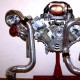TURBOCHARGER AIRFLOW MATH

Posted: August 10, 2011 in TurbochargersTo determine Absolute Pressure:

P1c + P2c = Absolute Pressure

P1c = Compressor Inlet Pressure

P2c = Compressor Discharge Pressure

Example:  14.7psia + 10psig = 24.7psi Absolute Pressure

To determine Pressure Ratio:

(P1c + P2c) / (P1c – 1) = Pressure Ratio

(Note: Any restriction in the air induction system caused by an air filter or ducting to the inducer side of a compressor creates a ‘depression’.  So, calculate for an approximate 1psig loss in the formula.  This is why ‘P1c – 1’ is used in the equation.)

Example: (14.7psia + 10psig) / (14.7psia – 1psig) = 1.80 Pressure Ratio

To determine the actual airflow needed to achieve a certain horsepower goal:

HP * A/F * (BSFC/60) = Wa

Wa = Airflow Actual (lbs/min)

HP = Desired Flywheel Horsepower

A/F = Desired Air/Fuel Ratio

BSFC/60 = Brake Specific Fuel Consumption divided by 60 to convert lb per hp/hr converted to minutes.

Example: 400 * 12 * (0.55/60) = 44.0 lb/min of air mass.

To determine Manifold Pressure required to meet flow target:

Wa  * R * (460 + Tm) / VE * (N/2) * Vd = MAPreq

MAPreq = Manifold Absolute Pressure required (psia) to meet airflow/horsepower target.

Tm = Intake Manifold Temperature (Degrees F)

VE = Volumetric Efficiency

N = Engine Rpm/ Speed

Vd = Engine Displacement in CID (cubic inch displacement)

Example: 44 * 639.6 * (460 + 130) / .92 * (7200/2) * 122 = 41.1 psi Absolute Pressure

So, 41.1 psi Absolute Pressure – 14.7 psia = 26.4 psig boost pressure

To determine Compressor Discharge pressure needed to compensate for pressure drop through induction system:

MAP + ∆Ploss = P2c

MAP = Manifold Absolute Pressure (psia)

∆Ploss = Pressure loss between the compressor outlet and the intake manifold (ex:2 psi)

P2c = Compressor Discharge Pressure

Example: 41.1 psia + 2 psi = 43.1 psia1.universalstang77 says:
•performancespecialties says: